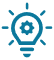# What is a boolean? How to use it in your program?

The boolean’s variable can take the values 0 (false) or 1 (true).

Each boolean variable use 1 Byte of memory :

• False (0000 0000)
• True (0000 0001)

Here is how we initialize a boolean:

``bool arduino_factory = true;``

The boolean can be use in if, for or while loop to express the condition we want to test.

We will see some examples:

``````bool variable =true;
void setup() {
Serial.begin(9600);
if (variable ==true){ // if the boolean is true
Serial.println("boolean true");
}
if (variable ==false){ // if the boolean is false
Serial.println("boolean false");
}
}

void loop() {
}``````

## Boolean expression

We will see now the different expressions that we can do with booleans.

a) Boolean expression OR

The boolean expression OR is used to verify if at least one of the both variables is at the state you wanted.

``````// We initialize the both boolean's variable
bool variable_1 = HIGH;
bool variable_2 = LOW;
void setup() {
Serial.begin(9600);
if (variable_1 == HIGH or variable_2 == HIGH){ // If one of the both variable is at the high state
Serial.println("variable 1 or 2 at high state");
}
else{ // If none of the both variable as a high state
Serial.println("variable 1 and 2 at a low state");
}
}
void loop() {
}``````

b) Boolean expression AND

The boolean expression AND can verify if the both variables are high state:

``````// We intialize declaration des deux variables booleens
bool variable_1 = HIGH;
bool variable_2 = LOW;
void setup() {
Serial.begin(9600);
if (variable_1 == HIGH and variable_2 == HIGH){ // If the both variables are at high state
Serial.println("variable 1 and 2 are at high state");
}
else{ // If at least one of the variable are not at high state
Serial.println("variable 1 or 2 at low state");
}
}

void loop() {
}``````

You can find others boolean expressions on our course on mathematics function.### How to reverse the state of a boolean?

We will see how to reverse the value (state) of the boolean with an exclamation point:

``````bool Variable_bool = true;
Variable_bool = !Variable_bool; // Reverse the stat therefore it's becoming false``````